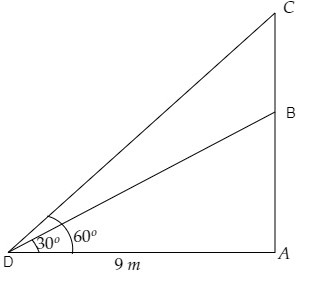# On a horizontal plane there is a vertical tower with a flag pole on the top of the tower. At a point 9 metres away from the foot of the tower the angle of elevation of the top and bottom of the flag pole are $60^{\circ}$ and $30^{\circ}$ respectively. Find the height of the tower and the flag pole mounted on it.

Given:

On a horizontal plane there is a vertical tower with a flag pole on the top of the tower.

At a point 9 metres away from the foot of the tower the angle of elevation of the top and bottom of the flag pole are $60^{\circ}$ and $30^{\circ}$ respectively.

To do:

We have to find the height of the tower and the flag pole mounted on it.

Solution:Let $AB$ be the tower and $BC$ be the flag pole on top of it.

Let $D$ be the point 9 metres away from the foot of the tower.

From the figure,

$\mathrm{AD}=9 \mathrm{~m}, \angle \mathrm{CDA}=60^{\circ}, \angle \mathrm{BDA}=30^{\circ}$

Let the height of the tower be $\mathrm{AB}=h \mathrm{~m}$ and the height of the flag pole be $\mathrm{BC}=x \mathrm{~m}$.

This implies,

$\mathrm{AC}=x+h \mathrm{~m}$

We know that,

$\tan \theta=\frac{\text { Opposite }}{\text { Adjacent }}$

$=\frac{\text { AB }}{DA}$

$\Rightarrow \tan 30^{\circ}=\frac{h}{9}$

$\Rightarrow \frac{1}{\sqrt3}=\frac{h}{9}$

$\Rightarrow h=\frac{9}{\sqrt3} \mathrm{~m}$

$\Rightarrow h=3\sqrt3 \mathrm{~m}$...........(i)

Similarly,

$\tan \theta=\frac{\text { Opposite }}{\text { Adjacent }}$

$=\frac{\text { AC }}{DA}$

$\Rightarrow \tan 60^{\circ}=\frac{h+x}{9}$

$\Rightarrow \sqrt3=\frac{3\sqrt3+x}{9}$                   [From (i)]

$\Rightarrow 9\sqrt3=3\sqrt3+x \mathrm{~m}$

$\Rightarrow x=(9-3)\sqrt3 \mathrm{~m}$

$\Rightarrow x=6\sqrt3 \mathrm{~m}$

Therefore, the height of the tower is $3\sqrt3 \mathrm{~m}$ and the height of the flag pole is $6\sqrt3 \mathrm{~m}$.

Updated on: 10-Oct-2022

26 Views